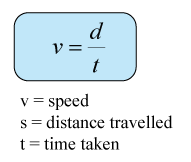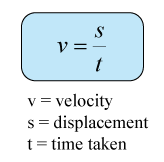# Speed and Velocity

Speed

1. Speed is defined as the rate of change in distance. It is a measure of how fast the distance change in a movement.
2. Speed is a scalar quantity.
3. The SI unit of speed is m/s (metre per second)

Equation of SpeedVelocity

1. Velocity is define as the rate of displacement change. It is the measure of how fast the displacement change of a moving object.
2. Velocity is a vector quantity.
3. The unit of velocity is m/s (metre per second)

Equation of velocityPositive or Negative Sign of Velocity